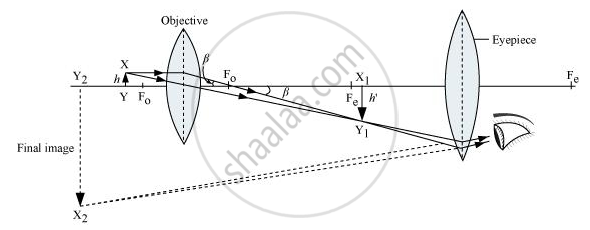# Draw the Labelled Ray Diagram for the Formation of Image by a Compound Microscope. Derive the Expression for the Total Magnification of a Compound Microscope. Explain Why Both the Objective and - Physics

#### Question

Draw the labelled ray diagram for the formation of image by a compound microscope.

Derive the expression for the total magnification of a compound microscope. Explain why both the objective and the eyepiece of a compound microscope must have short focal lengths.

#### SolutionShow L in figure

tan beta = h/(f_0)  .... (1)

tan beta = h'/L    ..... (2)

h'/h  =Lf_0    [Using (1) and (2)]

m_0 = h'/h = L/f_0 [Magnification due to objective]

m_e = 1+D/f_e

Net magnification (m) =m_0m_e

|m = (L/f_0) (D/f_e)|

f0 and fe are in denominator.

This formula contains foand fe in denominator. Therefore, both the objective and the eyepiece of a compound microscope must have short focal lengths.

Is there an error in this question or solution?

#### Video TutorialsVIEW ALL 

Draw the Labelled Ray Diagram for the Formation of Image by a Compound Microscope. Derive the Expression for the Total Magnification of a Compound Microscope. Explain Why Both the Objective and Concept: Optical Instruments - The Microscope.